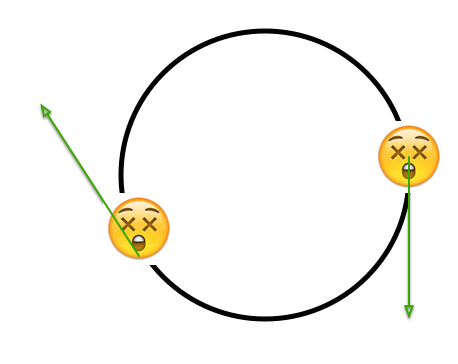# Uniform Circular MotionA particle is moving on a circular track with constant non-zero speed. Which of the following options are correct?

(a) The acceleration of the particle is zero.

(b) The rate of change of speed equals the magnitude of the rate of change of velocity.

(c) Instantaneous speed equals the magnitude of instantaneous velocity.

(d) The angle between velocity and acceleration has to be $90^\circ$.

×